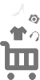### 搜索结果; //重试加载 var refreshLocation = function () { // var refreshedtimes = parseInt(\$.cookie('refreshedtimes')); // refreshedtimes += 1; // \$.cookie('refreshedtimes', refreshedtimes); // if (refreshedtimes <= 3) { setTimeout(window.location.reload(true), 1000);} // return false; } var to_language = 'zh_cn'; var page = 1; \$('.crumbs-bar em').html(resultjsonStr.total_results); //var page = {} //设置搜索结果到界面上 function setResult(json) { var str = ''; //\$("#search_product_result").children("img").get(0).hide(); \$(\$("#search_product_result").children("img").get(0)).hide(); var itemList = resultjsonStr.result_list.map_data; \$.each(itemList, function(key, item) { if(item.seller_id == '2759375209'){ return true;} //alert(1); var exchange_rate = 7.1; // var exchange_rate = ; //var dl=resultjsonStr.result_list.map_data; var price = (item.zk_final_price/exchange_rate).toFixed(2); str += '
• '; str += ''; str += '
￥ '+item.zk_final_price+' \$' +Math.round(item.zk_final_price/exchange_rate*100,2)/100 + '
'; str += '
'; str += ''+ item.title + ''; str += '
'; str += '
'; str += 'Seller : '+ item.nick +''; str += '
'; str += '
• '; }); //each //if(firstFlag == true){ // var beforeStr = ""; // \$("#search_product_result").empty(); //} else { // var beforeStr = \$('#search_product_result').html(); //} \$('#search_product_result').append(str); //goodsseller } var retry = 0; var rate=\$('#rate').html(); //汇率 //var htmlstr = ""; function handleData(json) { if (json.total_results == 0 || json.total_results==null || json.result_list.map_data == undefined) { \$("#search_product_result").empty(); return false; } else { setResult(json); } } //加载远程列表 var queryStr ="四件套"; var loadResult = function(cache_key){ if(queryStr == "" || queryStr == null){ return; } //var resultjsonjson = JSON.parse(resultjsonStr); handleData(resultjsonStr); }; //loadResutl loadResult(); });//document ready